Next: Shor's Algorithm for Quantum Up: Quantum Algorithms Previous: Quantum Algorithms   Contents

Subsections

Grover's Database Search

Many problems in classical computer science can be reformulated as searching a list for a unique element which matches some predefined condition. If no additional knowledge about the search-condition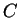is available, the best classical algorithm is a brute-force search i.e. the elements are sequentially tested againstand as soon as an element matches the condition, the algorithm terminates. For a list of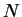elements, this requires an average of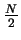comparisons.

By taking advantage of quantum parallelism and interference, Grover found a quantum algorithm which can find the matching element in only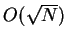steps. 

Formulating a Query

The most straightforward way, albeit not the most convenient for the algorithm, to implement the search condition is as a quantum function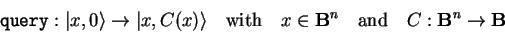(4.1)

as this allows us to formulate the problem within the realms of classical boolean logic.

Grover's algorithm can then be used to solve the equation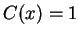while besides the fact that a solution exists and that it is unique, no additional knowledge about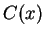is required.

Usually, the implementation of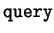will be complicated enough as not to allow an efficient algebraic solution, but since the inner structure ofdoesn't matter for the algorithm, we can easily implement a test query with the solution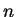as

 qufunct query(qureg x,quvoid f,int n) { int i; for i=0 to #x-1 { // x -> NOT (x XOR n) if not bit(n,i) { Not(x[i]); } } CNot(f,x); // flip f if x=1111.. for i=0 to #x-1 { // x <- NOT (x XOR n) if not bit(n,i) { !Not(x[i]); } } }

A more realistic application would be the search for an encryption key in a known-plaintext attack. With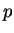being the known plaintext to the ciphertext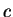, a QCL implementation could look like this:

 qufunct encrypt(int p,quconst key,quvoid c) { ... } qufunct query(int c,int p,quconst key,quvoid f) { int i; quscratch s[blocklength]; encrypt(p,key,s); for i=0 to #s-1 { // s -> NOT (s XOR p) if not bit(p,i) { Not(x[i]); } } CNot(f,x); // flip f if s=1111.. }
Note that, unlike the example above, this query function uses a local scratch register, so it isn't necessary to explicitely uncompute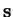, as this will be taken care of by QCL's internal scratch space management (see 3.3.1.5).

The Algorithm

Setting up the Search Space

The solution space of abit query conditionis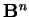. On a quantum computer, this search space can be implemented as a superposition of all eigenstates of anqubit register, i.e.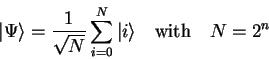(4.2)

In 3.5.1.1 we have shown how such a state can be prepared by a-qubit Hadamard transform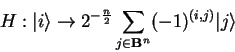(4.3)

(see 3.4.4.3) of the initial machine state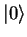.

The Main Loop

The main loop of the algorithm consists of two steps

1. Perform a conditional phase shift which rotates the phase of all eigenvectors which match the conditionby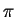radians.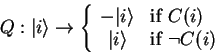(4.4)

2. Apply a diffusion operator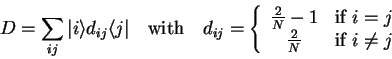(4.5)

Since only one eigenvector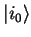is supposed to match the search condition, the conditional phase shift will turn the initial even superposition into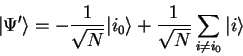(4.6)

The effect of the diffusion operator on an arbitrary eigenvector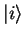is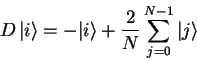(4.7)

so one iteration on a state of the form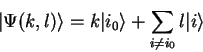(4.8)

amounts to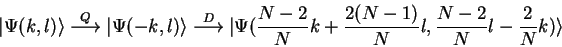(4.9)

Number of Iterations

If the above loop operator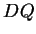is repeatedly applied to the initial superposition(4.10)

then the resulting states is still of the form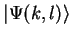and the complex amplitudes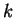and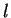are described by the following system of recursions: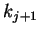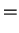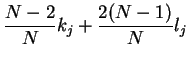(4.11)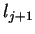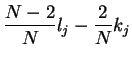(4.12)

Using the substitution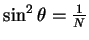the solution of the above system can be written in closed form.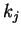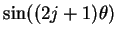(4.13)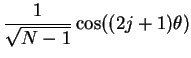(4.14)

The probabilityto measure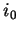is given as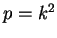and has a maximum at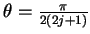. Since for large lists,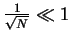we can assume that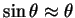and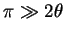and the number of iterations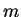for a maximumis about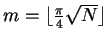with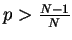(due to rounding errors). Alternatively, if we are content with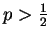, then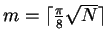iterations will do.

Implementation

The Query Operator

If we choose to formulate the query as quantum function with a flag qubit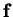to allow for a strictly classical implementation, as suggested in 4.1.1, then the operator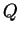can be constructed as(4.15)

by using the conditional phase gate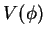(see 3.4.4.4) and considering the flag registeras temporary scratch space.

The Diffusion Operator

Using the Hadamard Transform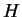(see 3.4.4.3) and a conditional phase rotation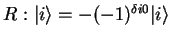, the diffusion operator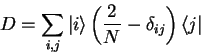(4.16)

can also be written as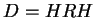since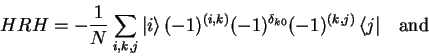(4.17)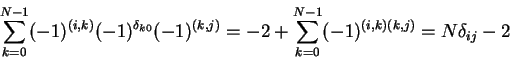(4.18)

Using the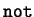operator from 3.4.7.4 and a conditional phase gatewe can implement the diffusion operator as

 operator diffuse(qureg q) { Mix(q); // Hadamard Transform Not(q); // Invert q CPhase(pi,q); // Rotate if q=1111.. !Not(q); // undo inversion !Mix(q); // undo Hadamard Transform }

In fact, the above operator implements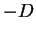, but since overall phases make no physical difference, this doesn't matter.

The Procedure grover

By using the above, we can now give a QCL implementation of the complete algorithm:

 procedure grover(int n) { int l=floor(log(n,2))+1; // no. of qubits int m=ceil(pi/8*sqrt(2^l)); // no. of iterations int x; int i; qureg q[l]; qureg f; { reset; Mix(q); // prepare superposition for i= 1 to m { // main loop query(q,f,n); // calculate C(q) CPhase(pi,f); // negate |n> !query(q,f,n); // undo C(q) diffuse(q); // diffusion operator } measure q,x; // measurement print "measured",x; } until x==n; }
The procedure argumentis the number to be found; the size of the quantum registers as well as the numbers of iterations are set accordingly:
 qcl> grover(500); : 9 qubits, using 9 iterations : measured 500 qcl> grover(123); : 7 qubits, using 5 iterations : measured 74 : measured 123 qcl> grover(1234); : 11 qubits, using 18 iterations : measured 1234Next: Shor's Algorithm for Quantum Up: Quantum Algorithms Previous: Quantum Algorithms   Contents

(c) Bernhard Ömer - oemer@tph.tuwien.ac.at - http://tph.tuwien.ac.at/~oemer/